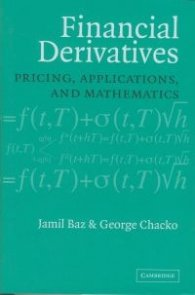# Financial Derivatives: Pricing, Applications, and MathematicsThis book offers a complete, succinct account of the principles of financial derivatives pricing. The first chapter provides readers with an intuitive exposition of basic random calculus. Concepts such as volatility and time, random walks, geometric Brownian motion, and Ito’s lemma are discussed heuristically.
The second chapter develops generic pricing techniques for assets and derivatives, determining the notion of a stochastic discount factor or pricing kernel, and then uses this concept to price conventional and exotic derivatives. The third chapter applies the pricing concepts to the special case of interest rate markets, namely, bonds and swaps, and discusses factor models and term structure consistent models. The fourth chapter deals with a variety of mathematical topics that underlie derivatives pricing and portfolio allocation decisions such as mean-reverting processes and jump processes and discusses related tools of stochastic calculus such as Kolmogorov equations, martingales techniques, stochastic control, and partial differential equations.

 Издател: Cambridge University Език: Английски Година: 2004 ISBN: 9780521815109 Страници: 350 Корици: твърди Тегло: 605 грама Размери: 23.8×16 Наличност: Не Раздел: Икономическа литература на чужди езици, Икономика, Книги

Цена: 133.00